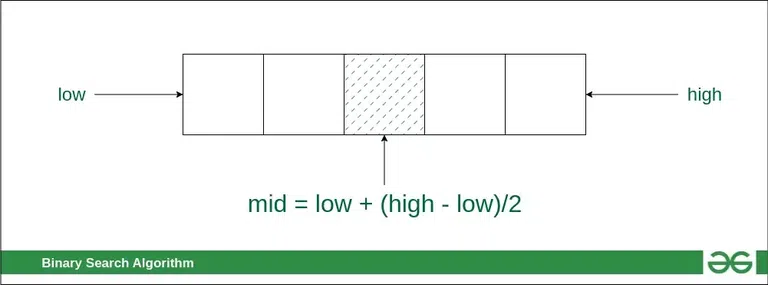GeeksforGeeks App
Open AppBrowser
Continue

# How to calculate “mid” or Middle Element Index in Binary Search?

The most common method to calculate mid or middle element index in Binary Search Algorithm is to find the middle of the highest index and lowest index of the searchable space, using the formula mid = low + \frac{(high – low)}{2}Finding the middle index “mid” in Binary Search Algorithm

## Is this method to find mid always correct in Binary Search?

Consider the following implementation of the Binary Search function:

## C

 // A iterative binary search function. It returns location// of x in given array arr[l..r] if present, otherwise -1 int binarySearch(int arr[], int low, int high, int x){    while (low <= high) {         // Find index of middle element        int mid = (low + high) / 2;         // Check if x is present at mid        if (arr[mid] == x)            return mid;         // If x greater, ignore left half        if (arr[mid] <= x)            low = mid + 1;         // If x is smaller, ignore right half        else            high = mid - 1;    }     // If we reach here, then element was not present    return -1;}

The above code looks fine except for one subtle thing, the expression

mid = (low + high)/2.

It fails for large values of low and high. Specifically, it fails if the sum of low and high is greater than the maximum positive value of int data type (i.e., 231 – 1). The sum overflows to a negative value, and the value stays negative when divided by two. This causes an array index out of bounds with unpredictable results.

### What is the correct way to calculate “mid” in Binary Search Algorithm?

The following is one way:

int mid = low + ((high – low) / 2);

Probably faster, and arguably as clear is (works only in Java, refer this):

int mid = (low + high) >>> 1;

In C and C++ (where you don’t have the >>> operator), you can do this:

mid = ((unsigned int)low + (unsigned int)high)) >> 1

A similar problem appears in other similar types of divide and conquer algorithms like Merge Sort as well. The above problem occurs when values of low and high are such that their sum is greater than the permissible limit of the data type. Although, this much size of an array is not likely to appear most of the time.

My Personal Notes arrow_drop_up